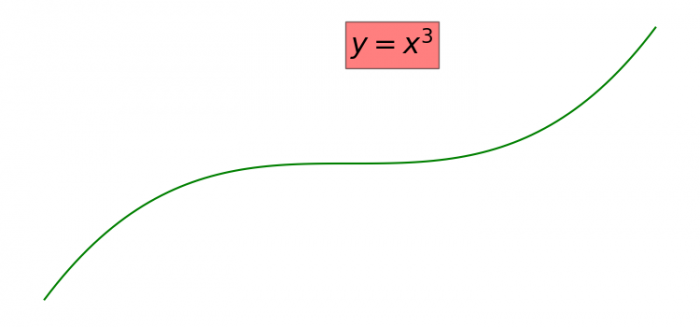# How to add text inside a plot in Matplotlib?

To add text inside a plot in Matplotlib, we can take the following steps −

• Set the figure size and adjust the padding between and around the subplots.
• Create x and y data points using numpy.
• Place text with some text properties.
• Plot x and y using plot() method.
• Turn off the axes.
• To display the figure, use show() method.

## Example

import numpy as np
from matplotlib import pyplot as plt

plt.rcParams["figure.figsize"] = [7.50, 3.50]
plt.rcParams["figure.autolayout"] = True

x = np.linspace(-5, 5, 100)
y = x ** 3

plt.text(0, 100, '$y=x^3$', fontsize=22, bbox=dict(facecolor='red', alpha=0.5))
plt.plot(x, y, c='g')
plt.axis('off')

plt.show()

## Output